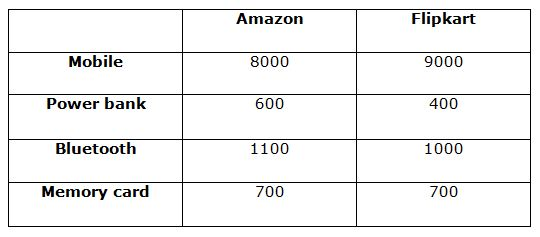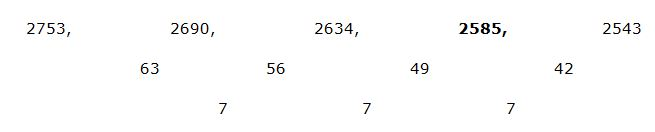# IBPS / LIC Clerk Prelims Quantitative Aptitude Questions 2019 (Day-05)

Dear Aspirants, Our IBPS Guide team is providing new series of Quantitative Aptitude Questions for IBPS Clerk Prelims 2019 so the aspirants can practice it on a daily basis. These questions are framed by our skilled experts after understanding your needs thoroughly. Aspirants can practice these new series questions daily to familiarize with the exact exam pattern and make your preparation effective.

Check here for IBPS Clerk Prelims Mock Test 2019

##### Check here for LIC Assistant Prelims Mock Test 2019
[WpProQuiz 7254]

Data Interpretation

Direction (1 – 5): Study the following information carefully and answer the questions given below.

The following Table shows the information about offered price of different items in Diwali sale of two online merchant Amazon and Flipkart.1) For mobile, in Flipkart and Amazon 20% and Rs. 700 discount is given. Then find the sum of original price of mobile?

a) 20000

b) 19590

c) 17950

d) 19950

e) 17850

2) For memory card, in Amazon and Flipkart 36% and 40% discount is given respectively. Then find the ratio of original price?

a) 8 : 5

b) 15 : 16

c) 5 : 8

d) 10 : 9

e) None of these

3) For Bluetooth in Amazon 12% discount is given and for power bank in Flipkart is sold at the 33.33% of the original price. Then the sum of offered price of power bank and Bluetooth in Amazon and Flipkart respectively is approximately what percentage of sum of original prices of Bluetooth in Amazon and power bank in Flipkart?

a) 65%

b) 75%

c) 120%

d) 150%

e) 90%

4) Find the ratio of average of offered price of all the items in Amazon and Flipkart?

a) 5: 4

b) 37: 19

c) 19: 37

d) 61: 37

e) None of these

5) Find the sum of original price of Mobile and power bank in Amazon and the offered price of Bluetooth and Memory card in Flipkart, if in Amazon the discount for all the product is 50 %?

a) 19800

b) 18900

c) 18790

d) 15780

e) 54780

Number series

Direction (Q. 6 – 10): What value should come in the place of (?) in the following number series?

6) 1259, 1288, ? , 1346, 1375

a) 1297

b) 1317

c) 1307

d) 1327

e) 1315

7) 2753, 2690, 2634, ? , 2543

a) 2564

b) 2574

c) 2587

d) 2622

e) 2585

8)  ?, 13377, 1911, 273, 39

a) 93639

b) 92279

c) 73279

d) 93539

e) 93489

9) 12, 10, 19, 51.5, ?

a) 97.5

b) 97.25

c) 64.5

d) 184.25

e) 87.25

10) 840, 960, 1120, 1344, ?

a) 1640

b) 1540

c) 1680

d) 1440

e) 1800

Directions (1-5) :

Original of mobile in,

Amazon = 8000 + 700 = 8700

Flipkart = 9000/80 * 100 = 11250

Required sum = 11250 + 8700 = 19950

Required ratio = [700/64 * 100]: [700/60 * 100]

= > 15 : 16

Original prices of Bluetooth in Amazon = 1100/88 * 100 = 1250

Original prices of power bank in Flipkart = 400/1 * 3 = 1200 (33.33% = 1/3)

Required % = [(1000 + 600)/(1250 + 1200)] * 100 = 65.3%

= 65%

Required Ratio = [(8000 + 600 + 1100 + 700)/4]: [(9000 + 400 + 1000 + 700)/4]

= 2600: 2775 = 104: 111

Required sum = (8000/1 * 2) + (600/1 * 2) + 1000 + 700

= > 16000 + 1200 + 1000 + 700 = 18900

Directions (6-10) :

1259           1288           1317          1346           1375

29               29               29               2993639/7 = 13377

13377/7 = 1911

1911/7 = 273

273/7 = 39

12 * 0.5 + 4 = 10

10 * 1.5 + 4 = 19

19 * 2.5 + 4 = 51.5

51.5 * 3.5 + 4 = 184.25

840 * 8/7 = 960

960 * 7/6 = 1120

1120 * 6/5 = 1344

1344 * 5/4 = 1680